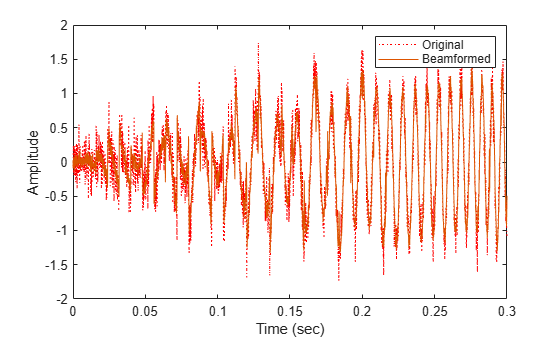# step

System object: phased.TimeDelayBeamformer
Package: phased

Perform time delay beamforming

## Syntax

```Y = step(H,X) Y = step(H,X,ANG) [Y,W] = step(___) ```

## Description

Note

Starting in R2016b, instead of using the `step` method to perform the operation defined by the System object™, you can call the object with arguments, as if it were a function. For example, ```y = step(obj,x)``` and `y = obj(x)` perform equivalent operations.

`Y = step(H,X)` performs time delay beamforming on the input, `X`, and returns the beamformed output in `Y`. `X` is an M-by-N matrix where N is the number of elements of the sensor array. `Y` is a column vector of length M.

The size of the first dimension of the input matrix can vary to simulate a changing signal length. A size change can occur, for example, in the case of a pulse waveform with variable pulse repetition frequency.

`Y = step(H,X,ANG)` uses `ANG` as the beamforming direction. This syntax is available when you set the `DirectionSource` property to`'Input port'`. `ANG` is a column vector of length 2 in the form of `[AzimuthAngle; ElevationAngle]` (in degrees). The azimuth angle must be between –180 and 180 degrees, and the elevation angle must be between –90 and 90 degrees.

`[Y,W] = step(___)` returns additional output, `W`, as the beamforming weights. This syntax is available when you set the `WeightsOutputPort` property to `true`. `W` is a column vector of length N. For a time delay beamformer, the weights are constant because the beamformer simply adds all the channels together and scales the result to preserve the signal power.

Note

The object performs an initialization the first time the object is executed. This initialization locks nontunable properties and input specifications, such as dimensions, complexity, and data type of the input data. If you change a nontunable property or an input specification, the System object issues an error. To change nontunable properties or inputs, you must first call the `release` method to unlock the object.

All input and output arguments can be single or double precision.

## Examples

expand all

Apply a time-delay beamformer to an 11-element uniform linear acoustic array. The arrival angle of the signal is -50 degrees in azimuth and 30 degrees in elevation. The arriving signal is a 0.3 second segment of a linear FM chirp having a 500 Hz bandwidth. Assume the speed of sound in air is 340.0 m/s.

Simulate the arriving signal at the wideband collector.

```microphone = phased.CustomMicrophoneElement('FrequencyVector',[20,20000],'FrequencyResponse',[1,1]); array = phased.ULA('Element',microphone,'NumElements',11,'ElementSpacing',0.04); fs = 8000; t = 0:1/fs:0.3; x = chirp(t,0,1,500); c = 340; collector = phased.WidebandCollector('Sensor',array,... 'PropagationSpeed',c,'SampleRate',fs,'ModulatedInput',false); incidentAngle = [-50;30]; x = collector(x.',incidentAngle);```

Add white gaussian random noise to the signal.

```sigma = 0.2; noise = sigma*randn(size(x)); rx = x + noise;```

Beamform the incident signals using a time-delay beamformer.

```beamformer = phased.TimeDelayBeamformer('SensorArray',array,... 'SampleRate',fs,'PropagationSpeed',c,... 'Direction',incidentAngle); y = beamformer(rx);```

Plot the beamformed signal against the incident signal at the middle sensor of the array.

```plot(t,rx(:,6),'r:',t,y) xlabel('Time (sec)') ylabel('Amplitude') legend('Original','Beamformed')```